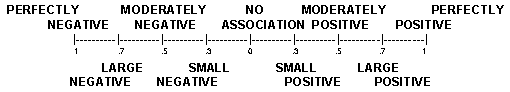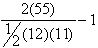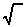previous lesson

Kendall's tau

ORDINAL DATA: Association and Inference

A. Kendall's Tau (data with ties): b = fa - fi
MV

Where:
fa = same as for gamma

fi = "          "   "      "

M = 1/2[N(N - 1)] - 1/2[ (row total)(row total - 1)]

V = 1/2[N(N - 1)] - 1/2 [ (column total)(column total - 1)]

Example: Data with ties:

Overall Happiness by Health

 EXCELLENT GOOD FAIR POOR VERY HAPPY 16 12 2 1 31 PRETTY HAPPY 15 29 11 3 58 NOT TOO HAPPY 1 5 4 2 12 32 46 17 6 101

SOURCE: GSS91 SURVEY SUBSAMPLE

Q: What is the association between overall happiness and health?

A: M = 1/2 (101)(100) - 1/2 [31(30) + 58(57) + 12(11)] = 2,866

V = 1/2 (101)(100) 1/2 [32(31) + 46(45) + 17(16) + 6(5)] = 3,368

b = 1,475 - 482 = .32
---(2,866)(3,368)

Interpretation: Use the scale below: "There is a ______ association between (variable 1) and (variable 2)."From example above: b = .32 = "moderately small positive association between health and happiness"

B. Tau (without ties on data):

1. Arrange first variable in order from smallest to largest. Rearrange second variable to correspond.

2. For each score on the second variable, its contribution to p (p is a variable representing place on list) is the number of scores located below it which are larger than it is.

3. Interpret same as for b: a = {2(P) / [1/2 N(N -1)]} - 1

Example:
 Person Occupational. Status Attractiveness Score P D 1 1 11 C 2 5 7 A 3 2 9 B 4 6 6 K 5 7 5 H 6 3 6 I 7 4 5 E 8 10 2 L 9 11 1 G 10 8 2 F 11 9 1 J 12 12 0 55=P

Q: What is the association between occupational status and attractiveness score?

A: a == 1.67 - 1 = .67 There is a moderately large positive assosiation between occupational status and attractiveness score.

C. Test of significance of Tau: Z = || / S

Where: S ("standard error of tau") = [2(2N + 5)] / [9N(N - 1)]

Assumptions: N 10

*P - value of Z on bottom in appendix.

Example: Using data above: occupational status/attractiveness score = .67, N=12

Q: Can this be generalized to the whole population?

A: S = 2(2(12) + 5) = .22 :-----Z = |.67 | = 3.0
---------9(12)(11) ------------------.22

Looking this up on p - value table, find p < .01; so yes, one can generalize.

D. Partial Tau:12.3=12 - (13)(23)

[1 - (13)2 ][1 -(23 )2 ]

Where:12 = tau between variables 1 and 2.13 = " " " 1 and 3.23 = " " " 2 and 3.

Example:
 Person Occ. Status Income Age A 1 5 5 B 2 3 3 C 3 4 4 D 4 1 2 E 5 2 1

Q: What is the association between occupational status and income with age held constant?

A: Variable held constant (age) is #3; let #1 = occupational status and #2 = income.12 = [2(2)] / [1/2(5)(4)] - 1 = -.6013 = -.8023  = +.8012.3 = (-.60) - [(-.80)(+.80)] =                             .04 = .11
---------[1 - (-.80)2 ][1 - (+.80)2 ]                     .36

Interpretation: Scale from -1 to +1, same as for Tau. From example: "Small positive relationship between occupational status and income with age held constant."

Congratulations!  You've completed all of the statistical work now for the SPSS project.